### Home | Up One Level | Previous | Next

WALTER RITZ

#### Annales 246 (Oeuvres 401)

In all that precedes, we have neglected the action of electrons on themselves; we are going to calculate it supposing the mass equal to M, the electric densities in two points xyz, x'y'z' being ε, ε'; furthermore, we will make abstraction of a rotation of the electron, and  consider only a quasi-stationary translation movement, that is to say where formula (13) for F* remains applicable. Formula (V) will then give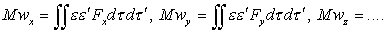The terms in u are nil in the expression of F, since the speed is the same for all elements of volume; the electrostatic term will again give zero, because it satisfies the principle of action and reaction; there remain only the terms relative to accelerations,

and we have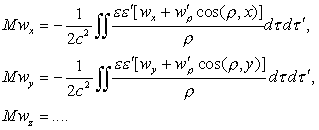The body will therefore exert on itself a force that is a linear function of the accelerations, it is to say a reaction of anisotropic inertia in general (that is to say dependent on the direction (Oeuvres 402) of w in relation to the electron):

(30)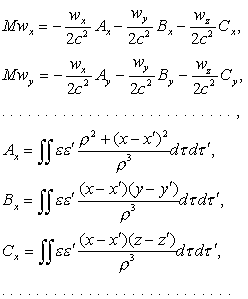where each combination of elements dτ, dτ' is taken twice.

These formulas are identical to those that result from Lorentz’s theory of low speeds; this is a result of the identity of the terms relative to accelerations in both theories.

When the body presents certain symmetries, this reaction becomes parallel to w; in this case, the x-axis being parallel to w, the quantity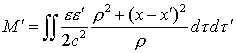will simply play the role of electromagnetic mass added to the mass M. Such is therefore the result of the inequality of the action and reaction in our theory for this particular case.

Besides, it is evident that this result subsists even at great speeds, as the terms in u will continue to be identically nil; the electromagnetic mass will not depend on speed in our theory and Kaufmann’s experiments should receive a new interpretation. Thus we will not be able to conclude from this that mass is of electromagnetic origin, but nothing stops us from admitting this hypothesis, at least for electrons.

Taking into account the terms in 1/c3, we obtain a new (Oeuvres 403) resultant force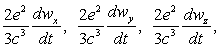that an electron of total charge e exerts on itself; we find it again, with the same coefficient, in Lorentz’s theory. This force, which must be added to the right side of equation (30), does not depend, as the inertia reaction does, on the form of the body, that is to say of the electron; it corresponds to a sort of friction determined by the loss of energy by radiation. In the solution of the equations where the term of the third order intervenes, it is only permissible to take into consideration the integrals corresponding to slow phenomena, for which developments in this series remain valid.

 Lorentz, Elektronentheorie, p.190. (Take into account the difference in units.

 Loc. cit.